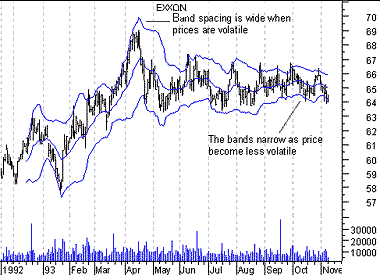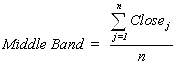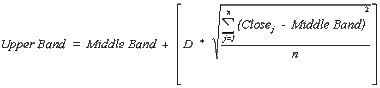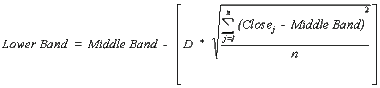# Technical Analysis from A to Z

by Steven B. Achelis

BOLLINGER BANDS®

Overview

Bollinger Bands are similar to moving average envelopes. The difference between Bollinger Bands and envelopes is envelopes are plotted at a fixed percentage above and below a moving average, whereas Bollinger Bands are plotted at standard deviation levels above and below a moving average. Since standard deviation is a measure of volatility, the bands are self-adjusting: widening during volatile markets and contracting during calmer periods.

Bollinger Bands were created by John Bollinger.

Interpretation

Bollinger Bands are usually displayed on top of security prices, but they can be displayed on an indicator. These comments refer to bands displayed on prices.

As with moving average envelopes, the basic interpretation of Bollinger Bands is that prices tend to stay within the upper- and lower-band. The distinctive characteristic of Bollinger Bands is that the spacing between the bands varies based on the volatility of the prices. During periods of extreme price changes (i.e., high volatility), the bands widen to become more forgiving. During periods of stagnant pricing (i.e., low volatility), the bands narrow to contain prices.

Mr. Bollinger notes the following characteristics of Bollinger Bands.

• Sharp price changes tend to occur after the bands tighten, as volatility lessens.

• When prices move outside the bands, a continuation of the current trend is implied.

• Bottoms and tops made outside the bands followed by bottoms and tops made inside the bands call for reversals in the trend.

• A move that originates at one band tends to go all the way to the other band. This observation is useful when projecting price targets.

Example

The following chart shows Bollinger Bands on Exxon's prices.The Bands were calculated using a 20-day exponential moving average and are spaced two deviations apart.

The bands were at their widest when prices were volatile during April. They narrowed when prices entered a consolidation period later in the year. The narrowing of the bands increases the probability of a sharp breakout in prices. The longer prices remain within the narrow bands the more likely a price breakout.

Calculation

Bollinger Bands are displayed as three bands. The middle band is a normal moving average. In the following formula, "n" is the number of time periods in the moving average (e.g., 20 days).The upper band is the same as the middle band, but it is shifted up by the number of standard deviations (e.g., two deviations). In this next formula, "D" is the number of standard deviations.The lower band is the moving average shifted down by the same number of standard deviations (i.e., "D").Mr. Bollinger recommends using "20" for the number of periods in the moving average, calculating the moving average using the "simple" method (as shown in the formula for the middle band), and using 2 standard deviations. He has also found that moving averages of less then 10 periods do not work very well.

This online edition of Technical Analysis from A to Z is reproduced here with permission from the author and publisher.